# Hydrophobicity Analysis of Amino Acids

by Arnold Neumaier, Waltraud Huyer and Erich Bornberg-Bauer

http://arnold-neumaier.at/software/protein/aminoacids.html

Abstract. Based on a principal component analysis of 47 published attempts to quantify hydrophobicity in terms of a single scale, we define a representation of the 20 amino acids as points in a 3-dimensional hydrophobicity space and display it by means of a minimal spanning tree. The dominant scale is found to be close to two scales derived from contact potentials.

The topological structure of the minimal spanning tree is shown in the following figure. The tree is labelled twice, by the standard three letter and one letter abbreviations for the amino acids.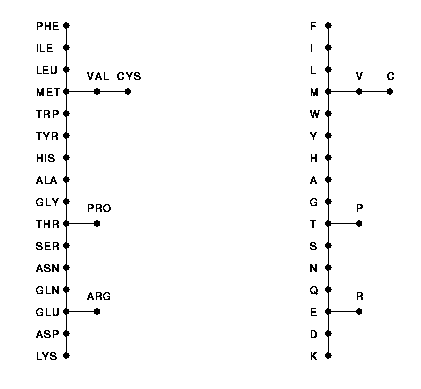Acknowledgment. The authors gratefully acknowledge partial support of this research by the Austrian Fonds zur Förderung der wissenschaftlichen Forschung (FWF) under grant P11516-MAT.

The assignment of the amino acids to a quantitative hydrophobicity scale is a controversial problem. A review and evaluation of 46 different scales is given in the paper

Sometimes, different scales differ widely already in the order in which the amino acids appear. This suggests that different scales measure different properties more or less directly related to hydrophobicity. The diversity of the scales then finds a natural explanation in the fact that amino acids cannot naturally be ordered in a linear way. However, a representation in higher-dimensional space might be possible in such a way that close amino acids have similar properties.

Restricting ourselves to those properties that are reflected in the known hydrophobicity scales, we performed a principal component analysis (see also here) of 40 scales, namely the 39 complete scales from the above survey (the 7 others are incomplete) and another scale (of so-called `q-values') from

Keeping only the three dominant principal components, we found the following coordinates of a three-dimensional representation of the 20 amino acids.

```
ALA   ARG   ASN   ASP   CYS
.06   .80   .70   .97  -.56
-.25   .19  -.06  -.08  -.40
.25  -.41   .17   .08  -.14

GLN   GLU   GLY   HIS   ILE
.71   .85   .32   .15 -1.00
-.02  -.10  -.32  -.03  -.03
.12  -.05   .28  -.10   .10

LEU   LYS   MET   PHE   PRO
-.83  1.00  -.68  -.99   .45
.05   .32  -.01   .18   .23
.01   .11   .04   .15   .41

SER   THR   TRP   TYR   VAL
.48   .38  -.57  -.35  -.75
-.15  -.10   .31   .40  -.19
.23   .29   .34  -.02   .03

```
The precise procedure was as follows: By a linear transformation we normalized each scale to mean 0 and standard deviation 1, then computed the singular value decomposition (also known as Karhunen-Loeve transform) and kept only the contributions to the three dominant scales x, y, and z. (The degree of explanation of the three scales, defined as the quotient of the corresponding squared singular value and the sum of squares of all singular values, was 75.7, 7.2, and 5.5 percent accounting for all but 11.6 percent of the information in all 40 scales.)
By another linear transformation we shifted the scales such their range was symmetric about zero, and multiplied all coordinates by the same constant such that x ranges between -1 and 1. We then rounded the values to two decimal places. (The large disagreement between the various scales implies that there is no point in representing the scales with higher accuracy; probably only the first figure is significant.)

It is not surprising that the first (dominant) coordinate x represents the bulk of the information in the 40 scales (75.7 percent). It can be considered to be most closely related to the amount of polarity or hydrophobicity, the common concept that all scales are supposed to measure.
Thus we regard x as the best compromise to a linear hydrophobicity scale. Polar amino acids have x>>0, hydrophobic ones have x<<0. Since between -.35 (TYR) and .06 (ALA), there is a large gap in the possible values for x, at least the classification into more or less polar amino acids and more or less hydrophobic ones is unambiguous.

The following figure represents the three scales by grey level bars (positive values are drawn dark, negative ones light) and in additions by marking the levels with crosses. The top scale contains x, the best compromise to a linear hydrophobicity scale. (The numbers are the positions of the amino acids in a lexicographic ordering.)A minimal spanning tree analysis (see also here) reveals that the appropriate nearest neighbor relation between amino acids is not fully linear. (The missing third dimension is indicated by the size of the markers; the fattest dots have a large positive missing coordinate.)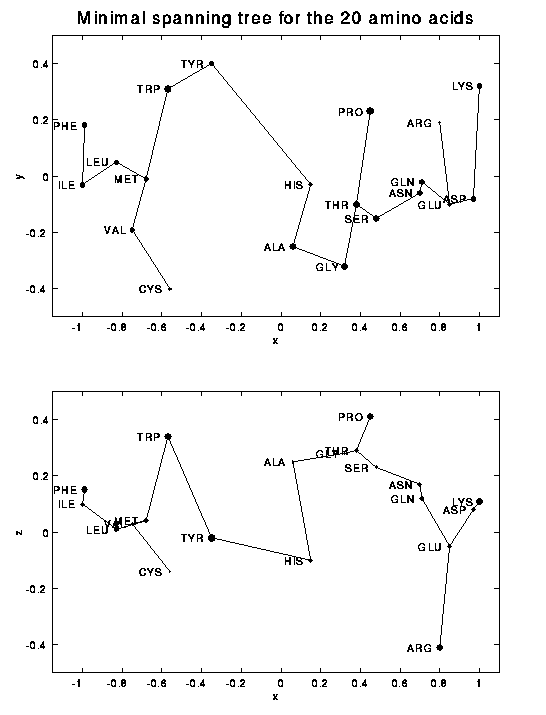The deviation from a linear ordering can also be seen by looking at a display of the distance matrix. Here the ordering has been chosen by appending the branches of the topological tree at the closer ends of the `backbone' of the tree. The distance is coded by grey values; dark entries correspond to close pairs.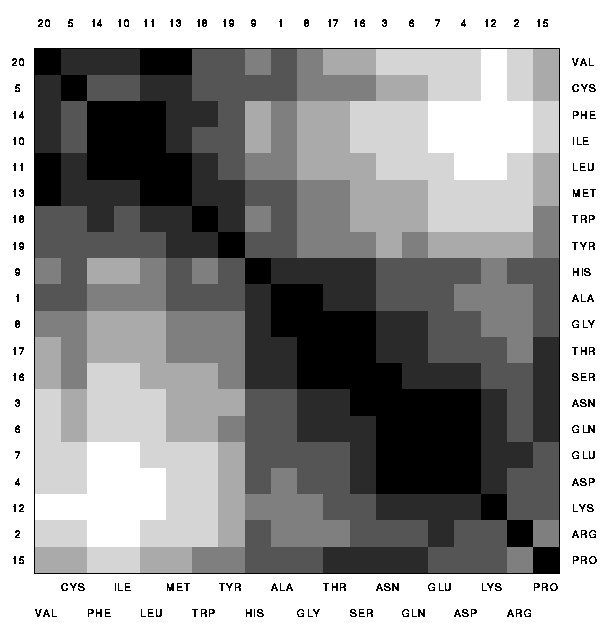Finally we consider how close a linear transformation of the original 47 scales is able to approximate the dominant scale x found above. We plotted each scale, linearly transformed to the range [-1,1], against x. (The 7 incomplete scales not used in the principal component analysis are marked with an asterisk\$^*\$.)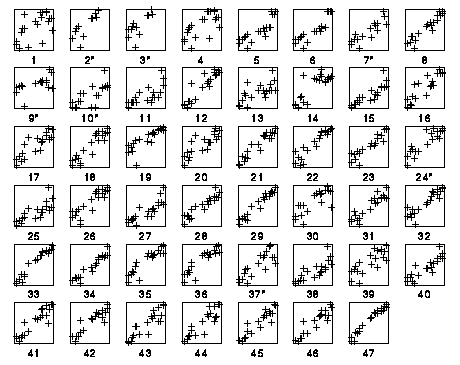As one easily sees from the plot, the scale that gives the best approximation to the dominant x scale (with a correlation of 0.982) is scale 47, the scale by Tang et al.. The other scales 1-46 correspond to Cornette et al. according to the following list. (Among these, the scale closest to x is scale 33=MIJER by Mijazawa and Jernigan, whose contact potentials were also used in a different way for the derivation of the Tang et al. scale.)

```
label   Cornette code        correlation with
x       y       z
1	EXP	ZIMMR       0.60   -0.42    0.13
2	EXP	N TAN       0.81   -0.84    0.10
3	EXP	NTANR       0.74   -0.71    0.04
4	EXP	JONES       0.69   -0.57   -0.10
5	X/S	LEVIT       0.84   -0.12   -0.37
6	X/S	HOPPW       0.87   -0.08   -0.30
7	EXP	YUNGD       0.86   -0.28   -0.30
8	EXP	FAUPL       0.94   -0.08   -0.20

9	EXP	ZASLZ       0.56   -0.58   -0.18
10	EXP	WOLF        0.72    0.40   -0.48
11	EXP	KUNTZ       0.69    0.18   -0.22
12	EXP	ABODR       0.92   -0.22   -0.15
13	EXP	MEEK        0.66   -0.47   -0.35
14	EXP	BULDG       0.81   -0.36    0.06
15	AVE	EISEN       0.86    0.18   -0.43
16	AVE	KYTDO       0.89    0.30   -0.12

17	STA	CHOTH       0.86    0.42   -0.11
18	STA	WERSC       0.92   -0.04    0.21
19	STA	JANIN       0.83    0.39    0.09
20	STA	OLSEN       0.82    0.40   -0.17
21	STA	MEIRO       0.95   -0.03    0.10
22	X/S	PONNU       0.93    0.17    0.24
23	STA	NNEIG       0.92    0.21    0.15
24	STA	ROBOS       0.88   -0.24    0.12

25	STA	CHDLG       0.78    0.40   -0.36
26	STA	WSDLG       0.88   -0.01    0.20
27	STA	JADLG       0.83    0.46   -0.22
28	STA	GUY         0.93    0.17   -0.04
29	AVE	GUY M       0.970   0.08    0.10
30	X/S	KRIDG       0.78   -0.44   -0.20
31	X/S	KRIGK       0.91    0.15    0.08
32	STA	NIOII       0.91    0.16    0.22

33	STA	MIJER       0.973   0.02    0.10
34	STA	ROSEF       0.96    0.18    0.09
35	STA	SWEET       0.91   -0.30    0.05
36	STA	SWEIG       0.91   -0.31    0.05
37	X/S	REKKR       0.82   -0.42    0.05
38	X/S	VHEBL       0.79    0.09   -0.52
39	X/S	FROMM       0.79   -0.55   -0.03
40	X/S	EIMCL       0.87   -0.21   -0.36

41	STA	PRIFT       0.91    0.04    0.34
42	STA	PRILS       0.91   -0.01    0.32

43	STA	ALFT        0.89   -0.06    0.27
44	STA	ALTLS       0.90   -0.03    0.29

45	STA	TOTFT       0.93    0.01    0.31
46	STA	TOTLS       0.92    0.00    0.32

47      TANG ET AL.         0.982  -0.08    0.05

```
Plotting the correlations for the various scales reveals a marked difference between experimental (EXP) and statistical (STA) scales. (Scales marked X/S are based on a mixture of experiment and statistics, and scales marked AVE are averages of other scales. The Tang et al. scale is marked STA.)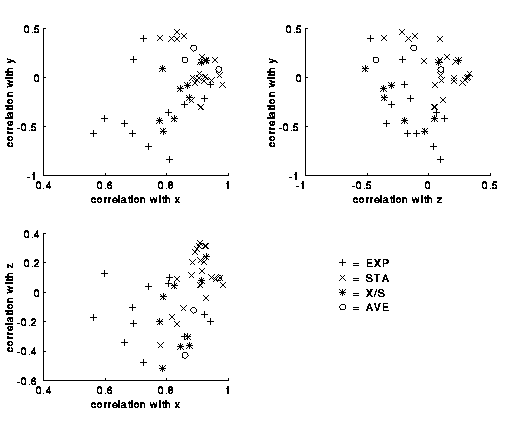Note, however, that the correlation coefficient is a quite generous measure of closeness of two scales. In particular, whether one transforms the Tang et al. scale (whose correlation coefficient 0.982 with x is best) linearly such that either (i) its mean and standard deviation agrees with x (see x' below) or (ii) the extremal values are at -1 and 1 (see x'' below), the scales don't match very closely:

```
x       x'      x''

0.06    0.16    0.25
0.80    0.59    0.65
0.70    0.58    0.64
0.97    0.79    0.84
-0.56   -0.42   -0.30
0.71    0.72    0.78
0.85    0.84    0.89
0.32    0.48    0.55
0.15    0.23    0.32
-1.00   -0.93   -0.79
-0.83   -1.16   -1.00
1.00    0.96    1.00
-0.68   -0.68   -0.55
-0.99   -1.13   -0.98
0.45    0.45    0.52
0.48    0.63    0.69
0.38    0.44    0.51
-0.57   -0.55   -0.43
-0.35   -0.26   -0.15
-0.75   -0.62   -0.50

```

The Amino Acids